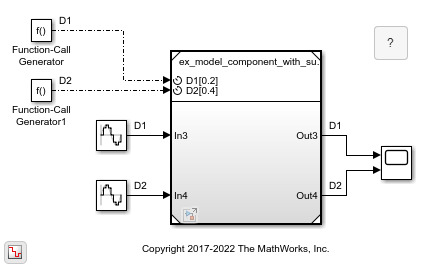## Test Rate-Based Model Simulation Using Function-Call Generators

To test a rate-based model, create a test harness for the rate-based model. The test harness is a model that references the rate-based model from a Model block. The Model block has periodic function-call event ports that receive function-call signals.

### Create Test Model That References Rate-Based Model

1. Add a Model block and configure it in the Property Inspector.

• Set Model name to the file name of a rate-based model. For example, use model `ex_rate_based_model` from Create Rate-Based Model.

• Under Model events simulation, select Schedule rates.

The Model block displays periodic function-call event ports.

2. Specify the execution rate using function-call initiators, such as Function-Call Generator blocks or Stateflow® charts. The function-call events and scheduling of the events are located outside of the Model block that references the rate-based model.

For example, add one Function-Call Generator block per periodic event port. Then, set the Sample time parameter of each Function-Call Generator block to match the sample time of the corresponding port.

Subsystems or referenced models in a rate-based model with the same sample time must have a common rate initiator. This requirement includes periodic scheduled subsystems and event-driven Function-Call Subsystem blocks with the same rate.

3. Use a fixed-step solver for simulation. On the Modeling tab, click . Then, in the Solver pane of the Configuration Parameters dialog box, set Type to `Fixed-step`.

4. Add source and sink blocks that connect to the input and output ports of the referenced model. For example, add Sine Wave and Scope blocks, respectively.

5. Optionally, add other controls, such as initialize, reinitialize, reset, and terminate functions to trigger events. For more information, see Using Initialize, Reinitialize, Reset, and Terminate Functions.

### Simulate Test Harness for Rate-Based Model

The `ex_model_test_harness_with_subsystems` model uses Function-Call Generator blocks to test the simulation of a rate-based model.The Model block references the rate-based model and schedules the rates of the model with periodic event ports. The generated function-call signals connect to the periodic event ports.

To observe the behavior of the rate-based model, simulate the test harness. Then, open the Scope block.### Avoid Common Compile and Run-Time Errors

Some common compile and run-time errors are caused by:

• A periodic event port that is not connected to a function-call initiator with the same specified sample time

• A scheduled Inport block (Sample time parameter set to a value) in the referenced model that does not specify one of the periodic function-call event port rates (sample times specified in the Port discrete rates table)